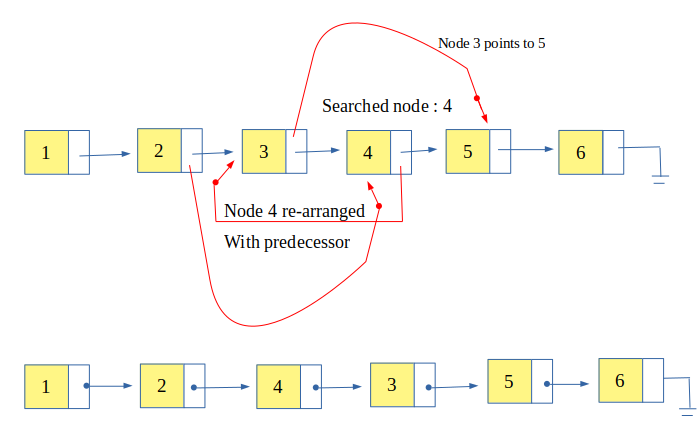# Self Organizing List : Transpose Method

Self Organizing list is a list that re-organizes or re-arranges itself for better performance. In a simple list, an item to be searched is looked for in a sequential manner which gives the time complexity of O(n). But in real scenario not all the items are searched frequently and most of the time only few items are searched multiple times.

So, a self organizing list uses this property (also known as locality of reference) that brings the most frequent used items at the head of the list. This increases the probability of finding the item at the start of the list and those elements which are rarely used are pushed to the back of the list.

In Transpose Method, the node that is accessed is swapped with its predecessor. So if any node is accessed, it is swapped with the node in-front of it unless it is the first(head) node in the list. In simple terms the priority of an accessed node is increased slowly such that it eventually reaches the first position. The main difference between transpose method and other methods is that, it takes many accesses to bring a node to front.Examples:

```Input :  list: 1, 2, 3, 4, 5, 6
searched: 4
Output : list: 1, 2, 4, 3, 5, 6

Input :  list: 1, 2, 4, 3, 5, 6
searched: 5
Output : list: 1, 2, 4, 5, 3, 6

Explanation: In 1st case, 4 is swapped with its predecessor i.e. 3
In 2nd case, 5 is swapped with its predecessor i.e. again 3
```

## Recommended: Please try your approach on {IDE} first, before moving on to the solution.

 `// CPP Program to implement self-organizing list ` `// using move to front method ` `#include ` `using` `namespace` `std; ` ` `  `// structure for self organizing list ` `struct` `self_list { ` `    ``int` `value; ` `    ``struct` `self_list* next; ` `}; ` ` `  `// head and rear pointing to start and end of list resp. ` `self_list *head = NULL, *rear = NULL; ` ` `  `// function to insert an element ` `void` `insert_self_list(``int` `number) ` `{ ` `    ``// creating a node ` `    ``self_list* temp = (self_list*)``malloc``(``sizeof``(self_list)); ` ` `  `    ``// assigning value to the created node; ` `    ``temp->value = number; ` `    ``temp->next = NULL; ` ` `  `    ``// first element of list ` `    ``if` `(head == NULL) ` `        ``head = rear = temp; ` ` `  `    ``// rest elements of list ` `    ``else` `{ ` `        ``rear->next = temp; ` `        ``rear = temp; ` `    ``} ` `} ` ` `  `// function to search the key in list ` `// and re-arrange self-organizing list ` `bool` `search_self_list(``int` `key) ` `{ ` `    ``// pointer to current node ` `    ``self_list* current = head; ` ` `  `    ``// pointer to previous node ` `    ``self_list* prev = NULL; ` ` `  `    ``// pointer to previous of previous ` `    ``self_list* prev_prev = NULL; ` ` `  `    ``// searching for the key ` `    ``while` `(current != NULL) { ` ` `  `        ``// if key found ` `        ``if` `(current->value == key) { ` ` `  `            ``// if key is neither the first element ` `            ``// and nor the second element ` `            ``if` `(prev_prev != NULL) { ` ` `  `                ``/* re-arranging the elements */` `                ``prev_prev->next = current; ` `                ``prev->next = current->next; ` `                ``current->next = prev; ` `            ``} ` ` `  `            ``// if key is second element ` `            ``else` `if` `(prev != NULL) { ` ` `  `                ``/* re-arranging the elements */` `                ``prev->next = current->next; ` `                ``current->next = prev; ` `                ``head = current; ` `            ``} ` `            ``return` `true``; ` `        ``} ` `        ``prev_prev = prev; ` `        ``prev = current; ` `        ``current = current->next; ` `    ``} ` ` `  `    ``// key not found ` `    ``return` `false``; ` `} ` ` `  `// function to display the list ` `void` `display() ` `{ ` `    ``if` `(head == NULL) { ` `        ``cout << ``"List is empty"` `<< endl; ` `        ``return``; ` `    ``} ` ` `  `    ``// temporary pointer pointing to head ` `    ``self_list* temp = head; ` `    ``cout << ``"List: "``; ` ` `  `    ``// sequentially displaying nodes ` `    ``while` `(temp != NULL) { ` `        ``cout << temp->value; ` `        ``if` `(temp->next != NULL) ` `            ``cout << ``" --> "``; ` ` `  `        ``// incrementing node pointer. ` `        ``temp = temp->next; ` `    ``} ` `    ``cout << endl ` `         ``<< endl; ` `} ` ` `  `// Driver Code ` `int` `main() ` `{ ` `    ``/* inserting five values */` `    ``insert_self_list(1); ` `    ``insert_self_list(2); ` `    ``insert_self_list(3); ` `    ``insert_self_list(4); ` `    ``insert_self_list(5); ` `    ``insert_self_list(6); ` ` `  `    ``// Display the list ` `    ``display(); ` ` `  `    ``// search 4 and if found then re-arrange ` `    ``if` `(search_self_list(4)) ` `        ``cout << ``"Searched: 4"` `<< endl; ` `    ``else` `        ``cout << ``"Not Found: 4"` `<< endl; ` ` `  `    ``// Display the list ` `    ``display(); ` ` `  `    ``// search 2 and if found then re-arrange ` `    ``if` `(search_self_list(5)) ` `        ``cout << ``"Searched: 5"` `<< endl; ` `    ``else` `        ``cout << ``"Not Found: 5"` `<< endl; ` `    ``display(); ` ` `  `    ``return` `0; ` `} `

Output:

```List: 1 --> 2 --> 3 --> 4 --> 5 --> 6

Searched: 4
List: 1 --> 2 --> 4 --> 3 --> 5 --> 6

Searched: 5
List: 1 --> 2 --> 4 --> 5 --> 3 --> 6
```

My Personal Notes arrow_drop_upCheck out this Author's contributed articles.

If you like GeeksforGeeks and would like to contribute, you can also write an article using contribute.geeksforgeeks.org or mail your article to contribute@geeksforgeeks.org. See your article appearing on the GeeksforGeeks main page and help other Geeks.

Please Improve this article if you find anything incorrect by clicking on the "Improve Article" button below.

Article Tags :
Practice Tags :

Be the First to upvote.

Please write to us at contribute@geeksforgeeks.org to report any issue with the above content.